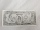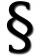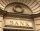# Loan

Apply for a $59000 loan, the loan repayment period is 8 years, the interest rate 7%. How much should I pay for every month (or every year if paid yearly). Example is for practise geometric progression and/or periodic payment for an annuity. ### Correct answer: m = 804.39 USD y = 9880.6 USD ### Step-by-step explanation:Did you find an error or inaccuracy? Feel free to write us. Thank you!Tips to related online calculators Do you want to convert time units like minutes to seconds? #### You need to know the following knowledge to solve this word math problem: ## Related math problems and questions: • InterestCalculate how much you earn for$n years $x deposit if the interest rate is$p% and the interest period is a quarter.
• Retirement annuityHow much will it cost to purchase a two-level retirement annuity that will pay $2000 at the end of every month for the first 10 years, and$3000 per month for the next 15 years? Assume that the payment represent a rate of return to the person receiving th
• Future valueSuppose you invested $1000 per quarter over a 15 years period. If money earns an anual rate of 6.5% compounded quarterly, how much would be available at the end of the time period? How much is the interest earn? • Present valueA bank loans a family$90,000 at 4.5% annual interest rate to purchase a house. The family agrees to pay the loan off by making monthly payments over a 15 year period. How much should the monthly payment be in order to pay off the debt in 15 years?
• JUDr. UsuryJudge JUDr. Usury approved the agreement on guilt and punishment where confessed to pay daily interest $d%. How big is a yearly interest? Year has$n days.
• Investment1000$is invested at 10% compound interest. What factor is the capital multiplied by each year? How much will be there after n=12 years? • Saving 9An amount of$ 2000 is invested at an interest of 5% per month. If $200 is added at the beginning of each successive month but no withdrawals. Give an expression for the value accumulated after n months. After how many months will the amount has accumula • LoanIf you take a bank loan$ 10000 and we want to repay after the year, we have to pay the total amount $10320/ What is the annual interest rate on this loan? • A loanA loan in the amount of$944 is charged simple interest at an annual rate of 8.1%. How much money is owed 14 months after the loan was made?
• SavingsThe depositor regularly wants to invest the same amount of money in the financial institution at the beginning of the year and wants to save 10,000 euros at the end of the tenth year. What amount should he deposit if the annual interest rate for the annua
• DepositIf you deposit 719 euros at the beginning of each year, how much money we have at 1.3% (compound) interest after 9 years?
• Compound interest 3After 8 years, what is the total amount of a compound interest investment of $25,000 at 3% interest, compounded quarterly? (interest is now dream - in the year 2019) • Annual interestA loan of 10 000 euro is to be repaid in annual payments over 10 years. Assuming a fixed 10% annual interest rate compounded annually, calculate: (a) the amount of each annual repayment (b) the total interest paid. • BankPaul put 10000 in the bank for 6 years. Calculate how much you will have in the bank if he not pick earned interest or change deposit conditions. The annual interest rate is 3.5%, and the tax on interest is 10%. • Simple interest 4Find the simple interest if$x USD at $p% for$m days. Assume a $r-day year. • If you 3If you deposit$4500 at 5% annual interest compound quarterly, how much money will be in the account after 10 years?
• Compound interestCompound interest: Clara deposited CZK 100,000 in the bank with an annual interest rate of 1.5%. Both money and interest remain deposited in the bank. How many CZK will be in the bank after 3 years?